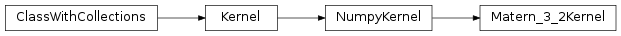# mvpa2.kernels.np.Matern_3_2Kernel¶class `mvpa2.kernels.np.``Matern_3_2Kernel`(length_scale=1.0, sigma_f=1.0, numerator=3.0, **kwargs)

The Matern kernel class for the case ni=3/2 or ni=5/2.

Note that it can handle a length scale for each dimension for Automtic Relevance Determination.

Attributes

 `descr` Description of the object if any

Methods

 `add_conversion`(typename, methodfull, methodraw) Adds methods to the Kernel class for new conversions `as_ls`(kernel) `as_np`() Converts this kernel to a Numpy-based representation `as_raw_ls`(kernel) `as_raw_np`() Directly return this kernel as a numpy array. `as_raw_sg`(kernel) Converts directly to a Shogun kernel `as_sg`(kernel) Converts this kernel to a Shogun-based representation `cleanup`() Wipe out internal representation `compute`(ds1[, ds2]) Generic computation of any kernel `computed`(\*args, \*\*kwargs) Compute kernel and return self `gradient`(data1, data2) Compute gradient of the kernel matrix. `reset`() `set_hyperparameters`(hyperparameter) Set hyperaparmeters from a vector.

Initialize a Squared Exponential kernel instance.

Parameters: length_scale : float or numpy.ndarray, optional the characteristic length-scale (or length-scales) of the phenomenon under investigation. (Defaults to 1.0) sigma_f : float, optional Signal standard deviation. (Defaults to 1.0) numerator : float, optional the numerator of parameter ni of Matern covariance functions. Currently only numerator=3.0 and numerator=5.0 are implemented. (Defaults to 3.0) enable_ca : None or list of str Names of the conditional attributes which should be enabled in addition to the default ones disable_ca : None or list of str Names of the conditional attributes which should be disabled

Attributes

 `descr` Description of the object if any

Methods

 `add_conversion`(typename, methodfull, methodraw) Adds methods to the Kernel class for new conversions `as_ls`(kernel) `as_np`() Converts this kernel to a Numpy-based representation `as_raw_ls`(kernel) `as_raw_np`() Directly return this kernel as a numpy array. `as_raw_sg`(kernel) Converts directly to a Shogun kernel `as_sg`(kernel) Converts this kernel to a Shogun-based representation `cleanup`() Wipe out internal representation `compute`(ds1[, ds2]) Generic computation of any kernel `computed`(\*args, \*\*kwargs) Compute kernel and return self `gradient`(data1, data2) Compute gradient of the kernel matrix. `reset`() `set_hyperparameters`(hyperparameter) Set hyperaparmeters from a vector.
`gradient`(data1, data2)

Compute gradient of the kernel matrix. A must for fast model selection with high-dimensional data.

`set_hyperparameters`(hyperparameter)

Set hyperaparmeters from a vector.

Used by model selection. Note: ‘numerator’ is not considered as an hyperparameter.